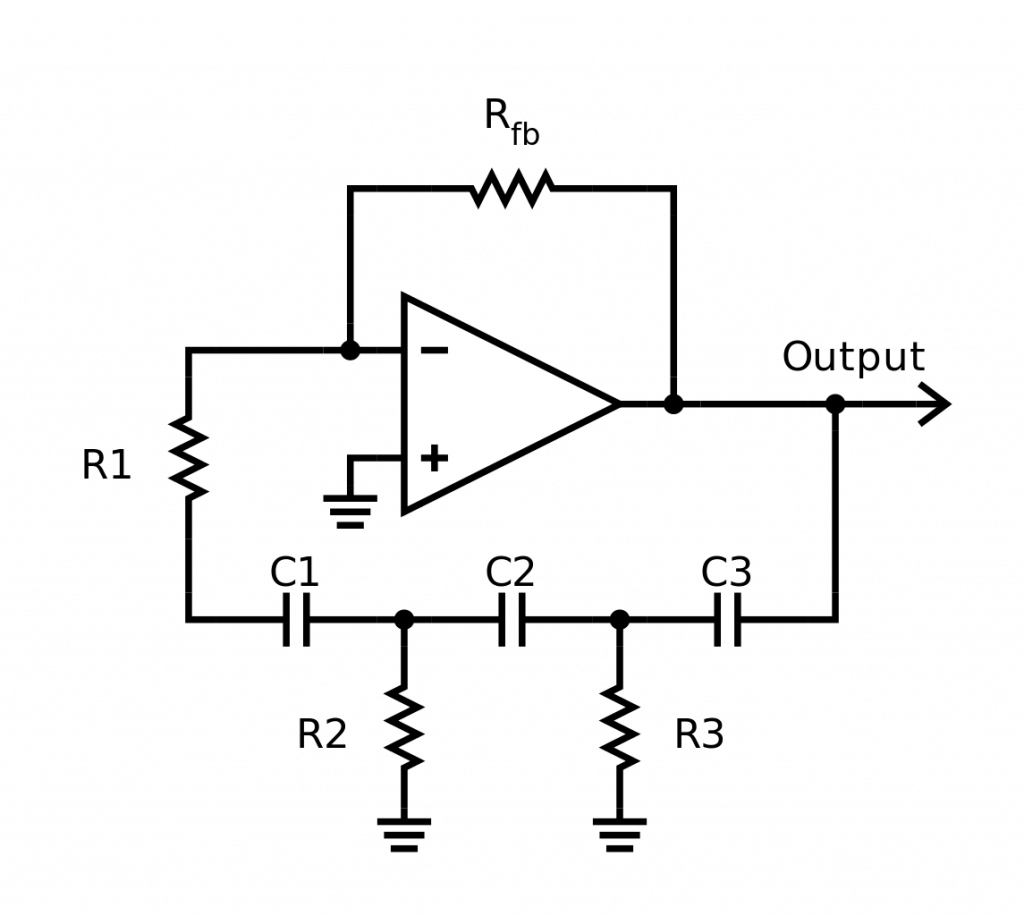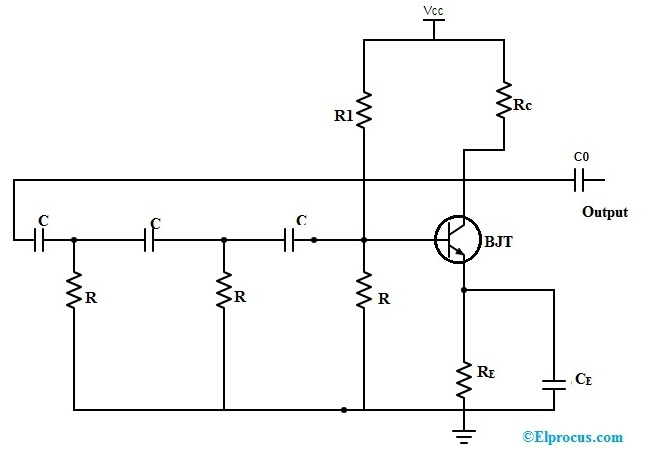# RC Phase Shift Oscillator Working and Its Applications

A phase shift oscillator can be defined as; it is one kind of linear oscillator which is used to generate a sine wave output. It comprises of an inverting amplifier component like operational amplifier otherwise a transistor. The output of this amplifier can be given as input with the help of the phase shifting network. This network can be built with resistors as well as capacitors in the form of a ladder network. The phase of the amplifier can be shifted to 1800 at the oscillation frequency by using a feedback network to provide a positive response. These types of oscillators are frequently used as audio oscillators on audio frequency. This article discusses an overview of RC phase shift oscillator.

## What is RC Phase Shift Oscillator?

RC phase-shift oscillator circuit can be built with a resistor as well as a capacitor. This circuit offers the required phase shift with the feedback signal. They have outstanding frequency strength and can give a clean sine wave for an extensive range of loads. Preferably an easy RC network can be expected to include an o/p which directs the input with 90o.RC Phase Shift Oscillator Circuit Diagram

But in actuality, the phase variation will be below this because the capacitor employed within the circuit cannot be perfect. Exactly the RC network’s phase angle can be expressed as

Ф= tan-1 Xc/R

In the above phase angle expression, XC can be 1/(2πfC), and it is the resistor & capacitor reactance. These kinds of networks offer a definite phase shift in oscillators.

The implementation and working of RC phase shift oscillator can be done using three methods namely RC phase shift oscillator using an op-amp, RC phase shift oscillator using BJT, and RC-phase shift oscillator using FET. For a better understanding of this concept here we are going to explain the following method.

## RC Phase Shift Oscillator Circuit Diagram using BJT

The following RC phase shift oscillator circuit using BJT can be built by cascading 3-RC phase shift networks; each provides a 600 phase shift. In the circuit, the RC which is known as the collector resistor stops the transistor’s collector current.

The resistor which is near to the transistors like R & R1 can form the voltage divider circuit as the RE (emitter resistor) develops the strength. After that, the two capacitors namely Co & CE, where Co is the o/p DC decoupling capacitor & CE is the emitter bypass capacitor correspondingly. Further, this circuit also demonstrates 3-RC networks used within the feedback path.RC Phase Shift Oscillator Circuit using BJT

This connection will cause the o/p waveform to move with 180o throughout its journey from o/p terminal toward the transistor’s base terminal. After that, this signal can be moved once more with 180o with the help of the transistor within the network because of the truth that the phase disparity among the input as well as the output can be 180o in the common emitter (CE) configuration. This will create the network phase disparity to 360 degrees and satisfies the phase disparity condition.

There is another method to satisfy the state of phase disparity is by using 4-RC networks, each provides a 450 phase shift. Therefore, the RC-phase shift oscillator is designed in different ways because the number of RC networks within them is unbalanced. But, by enhancing the number of stages will increase the circuit’s frequency strength; it also unfavorably influences the oscillator’s o/p frequency because of the loading effect.

### Frequency of RC Phase Shift Oscillator

The general equation for frequency of RC phase shift oscillator derivation can be expressed as

f= 1/2πRC√2N

Where,

R is the Resistance (Ohms)
C is the Capacitance
N is the no. of RC network

The above frequency formula can be used for High pass filter (HPF) related design, and can also be used LPF (low pass filter). In these cases the higher formula cannot work to calculate the oscillator frequency, another formula will be applicable.

Oscillator Frequency f= √N/2πRC

Where,

R is the Resistance (Ohms)
C is the Capacitance
N is the no. of RC network

### Advantages of RC Phase Shift Oscillator

The advantages of this phase shift oscillator include the following.

• The oscillator circuit designing is easy with basic components like resistors as well as capacitors.
• This circuit is not expensive and gives excellent frequency stability.
• These are mainly suitable for low-frequencies
• This circuit is simpler compared with a Wein bridge oscillator because it doesn’t require the stabilization planning & negative feedback.
• The circuit output is sinusoidal that is somewhat distortion free.
• The frequency range of this circuit will range from a few Hz to hundreds of kHz

### Disadvantages of RC-Phase Shift Oscillator

The disadvantages of this phase shift oscillator include the following.

• The output of this circuit is small because of the smaller feedback
• It requires 12 volts battery for developing a suitably huge feedback voltage.
• It is hard for this circuit to create oscillations because of the small feedback
• The frequency stability of this circuit is not good to compare with Wien bridge oscillator.

### RC Phase Shift Oscillator Applications

The applications of this type of phase shift oscillator include the following

• This phase shift oscillator is used to generate the signals over an extensive range of frequency. They used in musical instruments, GPS units, & voice synthesis.
• The applications of this phase shift oscillator include voice synthesis, musical instruments, and GPS units.

Thus, this is all about RC phase shift oscillator theory. From the above information finally, we can conclude that these oscillators are mainly used to generate signals in the wide range. The range of frequency can be changed from Hz-200Hz by using resistors as well as capacitors. Here is a question for you, what is the main function of phase shift oscillator?